# Rainbow

A rainbow occurs when you have both sunlight and rain. The following is an attempt to explain why you see the colours and where the rainbow is formed in the sky.Figure 1 shows a ray of light from the Sun being partially internally reflected by a spherical raindrop. (We will have to ignore the tiny deformation of the drop due to gravitational attraction). Notice that rainbows are NOT due to total internal reflection. If they were the light would never be able to escape from the spherical water drop. The amount of light reflected will vary with the deviation but will be maximum at, or near minimum deviation.

The path of a ray of light through a spherical raindrop is shown in Figure 1. (Note that the transmitted light at C has not been shown as it does not contribute to the rainbow).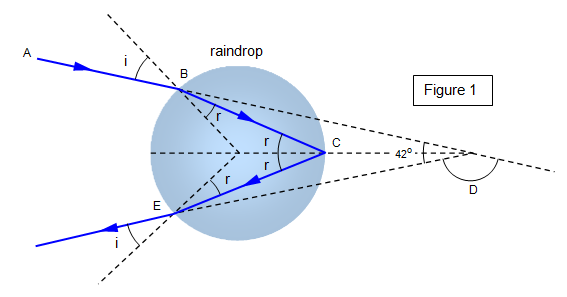For one internal reflection (the primary rainbow) we have: D= 180 +2i - 4r

Also for minimum deviation (dD/di = 0) we can show that: cos i = ([n-1]/3)1/2
where n is the refractive index of the water of the raindrop.

The deviation for yellow light (n = 1.34) is about 138o and so the observer will see a rainbow in a direction that makes 180° - 138° = 42° with the Sun's altitude (see Figure 2(a) and (b)).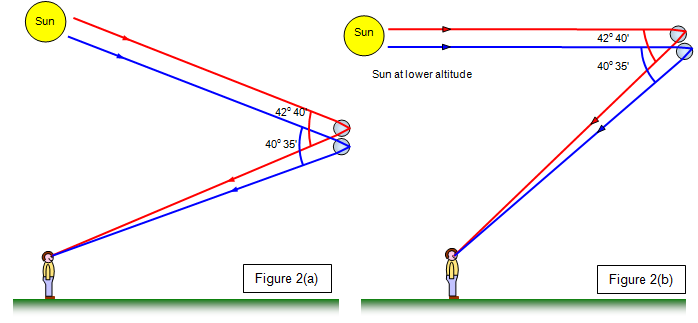To see the rainbow you would need to be facing the area of the sky with the rain in it with your back towards the Sun. (Figure 3).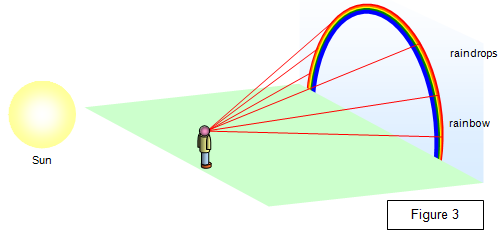### A circular rainbow

If you were up in a plane or standing on a mountain it would be possible to see a circular rainbow – the ground would not 'get in the way' of the lower half of the circle. I have seen one of these from a small plane over the South Pacific.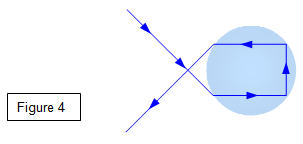### A secondary rainbow

For a secondary rainbow to be formed the light must make two internal reflections inside the raindrop as shown in Figure 4.### Our own personal rainbow

We all see our own rainbow and if we walk around it moves with us. You should be able to see why this is true by looking at Figures 5 and 6.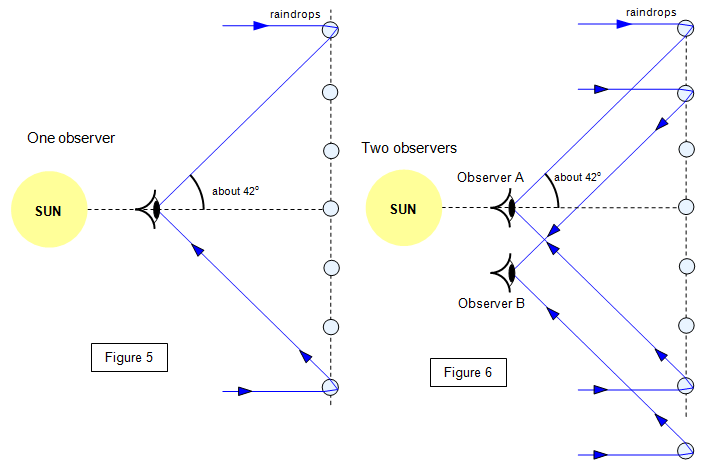For a full proof the formulae see 'A Second Course of Light' - A.E.Mckenzie CUP 1962

A VERSION IN WORD IS AVAILABLE ON THE SCHOOLPHYSICS USB